On the Scalability of Social Order: Modeling the Problem of Double and Multi Contingency Following Luhmann

Journal of Artificial Societies and Social Simulation vol. 6, no. 1
<https://www.jasss.org/6/1/3.html>

To cite articles published in the Journal of Artificial Societies and Social Simulation, please reference the above information and include paragraph numbers if necessary

Received: 14-Jun-2002      Accepted: 9-Jan-2003      Published: 31-Jan-2003

###Abstract

We investigate an algorithmic model based first of all on Luhmann's description of how social order may originate [N. Luhmann, Soziale Systeme, Frankfurt/Main, Suhrkamp, 1984, pp. 148-179]. In a basic 'dyadic' setting, two agents build up expectations during their interaction process. First, we include only two factors into the decision process of an agent, namely, its expectation about the future and its expectation about the other agent's expectation (called 'expectation-expectation' by Luhmann). Simulation experiments of the model reveal that 'social' order appears in the dyadic situation for a wide range of parameter settings, in accordance with Luhmann. If we move from the dyadic situation of two agents to a population of many interacting agents, we observe that the order usually disappears. In our simulation experiments, scalable order appears only for very specific cases, namely, if agents generate expectation- expectations based on the activity of other agents and if there is a mechanism of 'information proliferation', in our case created by observation of others. In a final demonstration we show that our model allows the transition from a more actor oriented perspective of social interaction to a systems-level perspective. This is achieved by deriving an 'activity system' from the microscopic interactions of the agents. Activity systems allow to describe situations (states) on a macroscopic level independent from the underlying population of agents. They also allow to draw conclusions on the scalability of social order.

Keywords:
Artificial Chemistry; Coordination; Double Contingency; Learning; Networks; Self-organization; System Theory

###Introduction

1.1
How is social order possible? This is one of the most fundamental question of sociology since its beginning. Several answers have been given in the last 350 years, such as: social order is generated by a powerful state, the Leviathan (Hobbes 1651); by an "invisible hand" (Smith 1776); by norms (Durkheim 1893), which are legitimated by values located in a cultural system of a society (Parsons 1937; Parsons 1971); or by rational choice of action in consideration of a long common future (shadow) (Axelrod 1984).

1.2
Another prominent proposal refers to the problem of double contingency. Parsons (1968, p. 436), has formulated this problem as follows: "The crucial reference points for analyzing interaction are two: (1) That each actor is both acting agent and object of orientation both to himself and to the others; and (2) that, as acting agent orients to himself and to others, in all of primary modes of aspects. The actor is knower and object of cognition, utilizer of instrumental means and himself a means, emotionally attached to others and an object of attachment, evaluator and object of evaluation, interpreter of symbols and himself a symbol."

1.3
Following Parsons (1968), Luhmann (1984) identified the problem of double contingency as the main problem of producing social order. The problematic situation is this: two entities meet each other. How should they act, if they want to solve the problem of contingency, that is, if necessities and impossibilities are excluded?

1.4
Parsons' solution for this problem - a consensus on the basis of a common shared symbol system - was strongly criticized, because the question how a common shared symbol system can develop before social order emerges cannot be answered any longer in the context of the situation of double contingency. As Parsons admits: "This is one sense in which the dyad is clearly a limiting case of interaction. However isolated a dyad may be in other respects, it can never generate the ramified common culture which makes meaningful and stable interaction possible. A dyad always presupposes a culture shared in a wider system. Furthermore, such a culture is always the product of a historical process long transcending the duration of a particular dyadic relationship." (Parsons 1968, p. 437).

1.5
Luhmann's assumptions for the solution of the problem of double contingency are more basic, in so far as he searches for a solution not in the social dimension as the consensus would be, but in self-organization processes in the dimension of time. In a first step an entity would begin to act tentatively, e.g., with a glance or a gesture. Subsequent steps referring to this first step would be contingency reducing activities, so that the entities would be able to build up expectations. As a consequence, a system history develops. Beginning from this starting point further mechanisms could be instituted to generate order, such as confidence or symbolic generalized media. Thus, social structures, social order or social systems are first of all structures of mutual expectations. That is, every entity expects that the other entity has expectations about its next activity.

1.6
In this paper we model and simulate the situation of double contingency as the origin of social order. We shall concentrate on specific aspects of fundamental order formation by beginning with an actor-theoretical framework as formulated by Parsons. The actor-theoretical approach allows the transition to a multi-agent system and to switch to a system level perspective.

1.7
Notably, we do not consider approaches like those including aspects of rationality (Lepperhoff 2000) or game theory (Lomborg 1996; Taiji and Ikegami 1999). Instead, we model and analyze the way of producing "social" order, from the basic assumptions of the situation of double contingency: a dialectic constellation, mutual inscrutableness, necessity of expectation-expectation and expectation-certainty and no external assumptions, e.g., norms or values.

###The Model

2.1
The model consists of agents exchanging messages. The basic model is dyadic and restricted to two agents called A and B, or Ego and Alter, respectively. There are N different messages used and recognized by the agents. There is no a priori relationship between messages. Two agents interact only by exchanging message. Messages are exchanged alternatively. This means that Agent A sends one message out of N possible messages. After receiving this message, Agent B sends one message on his part; and so on.

2.2
We can imagine that each agent displays the message he would like to send on a sign he holds Figure (1). In our case, the message is just a number written on the sign. An activity of an agent consists of changing the number on his sign after observing the sign of the other agent.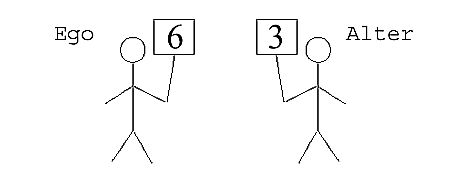Figure 1. Two agents interact by showing signs symbolizing messages.

### Motivation and Activity Selection

2.3
What are the agents' motives that influence the selection of a specific activity? Selecting and performing activity i is equivalent to displaying a particular number i on the sign. Here, we consider two fundamental motives: (1) expectation-expectation (EE): an agent wants to meet the expectations of the other agent, (2) expectation-certainty (EC): the reaction of the other agent following its own activity should be as predictable as possible. Note that both motives might contradict each other.

2.4
Agent A selects an activity in the following way: (1) For each activity i it determines how much i is expected by Agent B (expectation-expectation). (2) It then determines how well the reaction of Agent B following activity i can be predicted (expectation-certainty). (3) It combines both values by a weighted sum in order to arrive at the activity value. Activity values are calculated "weights" for each possible activity that are used to select an activity: The larger an activity value, the more probable that the corresponding activity is selected. The impact of randomness can be easily varied in our model by adjusting a parameter called γ between 0 (maximum randomness) and ∞ (maximum determinism), as can be seen by Eq. 3 below.

2.5
A more precise description follows:

1. For each possible activity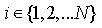compute:

(a) expectation-expectation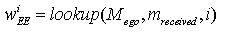Here, an agent estimates the probability that Agent B expects activity i to be performed next by Agent A. The value is determined by accessing A's memory Mego which has stored responses of Agent A to activities of Agent B. mreceived is the last activity of Agent B to which Agent A has to respond now. In other words, mreceived is the number that Agent B displays on its sign. Roughly speaking, the function lookup(Mego, mreceived, i) returns how often Agent A has reacted with activity i to activity mreceived in the past. In order to model forgetting, past events long passed are counted less than recent events (see Section 2.12, below).

(b) expectation-certaintyIn order to calculate the certainty of the future, Agent A possesses a second memory Malter, which stores how the other agent has reacted to A's activities. So, using this memory, A can predict what B might do as a response to A's potential activity i. Consulting A's alter-memory by calling the function lookup(Malter, i) results in a vector (p1, ...,pN) containing N values. A value pj ∈ [0; 1] of this vector is an estimate of the probability that Agent B responds with activity j to activity i of Agent A. The expectation-certainty is measured by the function fcertainty. The input of that function is the vector (p1,...,pN). The function fcertainty returns a certainty of 0.0, if all values of the vector are the same, e.g., (1/N, 1/N, ..., 1/N), since in that case the agent has no information (there is no distinction possible). The highest certainty (value 1.0) is returned for a vector that consists of zeros but a single value 1, e.g., (1, 0, 0, ..., 0). For this work we measure the certainty by the Shannon entropy (Shannon and Weaver 1949):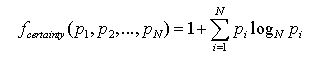(1)

(See Section 9.5 in the Appendix for alternative certainty measures.)

(c) activity valueThe expectation-expectation wiEE and the expectation-certainty wiEC are combined by function f and the result is normalized in order to calculate the activity value wiAV. The parameter α specifies the fraction of EC contained in the activity value. f is a linear sum plus a small additive constant:(2)

with cf being a constant parameter. The addition of cf / N prevents an activity value from approaching zero in order to avoid artefacts. Division by N assures that the influence of the constant summand does not increase with increasing N. For the experiments presented here we have chosen cf = 0.01 so that there is always at least a small chance for each activity to be selected. In a situation where an agent is rather sure what to do and proportional selection (explained below) is used, there is a chance of about 1% that an activity is selected different from the most probable one. In case of quadratic selection (γ= 2) the "error" probability caused by cf is about 0.2%. (See Section 9.1 in the Appendix for a detailed discussion of cf.)

2. activity probabilities wAP=g(wAV)
The activity values are scaled by the selection function g: RN → RN: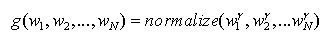(3)

The parameter γ allows to control the influence of randomness (see below). Note that y is an exponent in Eq. (3).

3. Randomly select activity i such that the probability of activity i is wiAP

2.6
As indicated, selection is controlled by a parameter y. The larger y the smaller the influence of randomness. For simplification we have defined the following selection methods based on different choices for y:

• Maximizing selection (γ = ∞ ): Choose activity i with largest activity value wiAV. (The rational choice.)
• Proportional selection (γ = 1): Randomly choose activity i such that the probability of i is proportional to activity value wiAV.
• Quadratic selection (γ = 2): Randomly choose activity i such that the probability of i is proportional to the activity value wiAV squared

#### The Memory and Prediction Component

2.7
Our agents possess memory in order to store observed events. Stored observations are subsequently used to predict future events. The ability to predict future events is necessary in order to build up expectations, which are an important component of Luhmann's description of the genesis of social order (Luhmann 1984, Chapter 4).

2.8
Note that forgetting is an important feature of the memory. Only if agents forget events, they free capacity for new situations. If they would store everything, the capacity of information processing would run down quickly or the simulation experiments would require unproportional computational resources. So, memorized objects emerge by the repression of forgetting. One can say that the memory in general connects activities.

2.9
Our agent implementation has been designed with two memory modules for each agent, one for storing its own responses and one for storing the other agent's responses. We will call these memory modules ego-memory Mego and alter-memory Malter, respectively. An event to be stored in memory, is a pair (a,b) ∈ M 2 where a ∈ M is a message (or activity) and b is the response to a, M = {1, 2,..., N}.

2.10
We formalize the memory as an abstract data type called Memory with the following interface functions: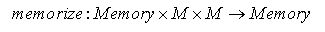(4)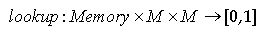(5)

2.11
The function memorize stores an event in memory. Given a memory M ∈ Memory, calling lookup(M, a, b) returns the estimated probability that the event (a, b) will be observed, e.g., the estimated probability that an agent responds to activity a with activity b. Mathematically, we demand that the output of lookup is a quantity with features of a probability, i.e., normalized for each memory M: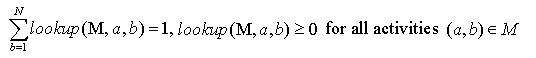(6)

In order to simplify the following formalism we define a function which returns a vector of all estimated probabilities for every possible response to message a: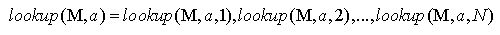(7)

#### The Simple Neuronal Memory

2.12
In the previous section we have described the memory as an abstract data type by specifying interface functions and their general meaning. In our simulation software many different memory models are implemented (see Section 9.4 in the Appendix), with each possessing the same interface functions. In this contribution a simple neuronal memory is used as defined by:

Representation: A memory M is represented by a dimensional matrix ma,b called memory matrix. This matrix (ma,b) is manipulated by the following initialization, memorization and lookup procedures:

Initialization: The matrix is initialized with (ma,b) = 1/N.

Memorize(M, a, b): First, we increase the entry in the memory matrix given by the index (a, b) by the learning rate rlearn :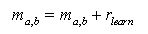(8)

Then we increase all entries by the forgetting rate rforget divided by the number of activities N:(9)

Finally we normalize every line of the memory matrix:(10)

Lookup(M, a, b): Return the entry of the memory matrix given by index (a, b):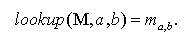(11)

#### Example

2.13
As an example we take a look at the first three steps of a simulation experiment with the following settings: Two agents (dyadic world scenario), N = 2 different activities, normal learning rate rlearn = 0.1 and forgetting rate rforget = 0,01, EE-EC ratio a = 0.5, quadratic selection method, γ = 2.

2.14
After initialization the state of Agent A looks like:2.15
Note that the "presented message on sign" is initialized randomly. Here, by chance it is initialized with activity 1 in both cases.

2.16
Now Agent A has to select an activity. The activity of Agent B (equal to the number on the sign presented by Agent B) has been activity 1. So, A has to react to activity 1.

2.17
For this purpose a couple of calculations have to be performed: First, A tries to estimate, what kind of activity Agent B expects from him. A is doing this estimate by consulting his (A's) ego-memory:Second, the expectation-certainty is calculated by using the alter-memory: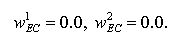2.18
This means, that Agent A has no information about what can happen once he performs activity 1 or activity 2. Practically, these two values are calculated by taking the entropy of the two rows of the alter-memory. Recall that the first row of the alter-memory represents the estimated probabilities describing how the other agent might react to activity 1 and the second row how he might react to activity 2. Next, EE and EC are combined by function f: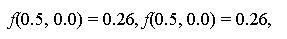and the result is normalized to arrive at the activity values: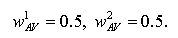2.19
Finally, the activity values are scaled by the function g in order to get activity probabilities. In our example, this action will not change anything, since both activity values are the same. So we have: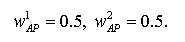2.20
We can summarize the decision process of Agent A as follows: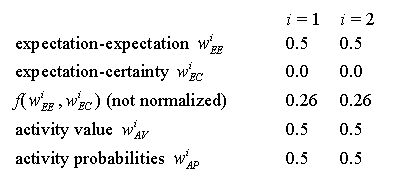2.21
In this situation, A selects an activity randomly, since the probability is the same for each activity. In our example, Agent A selects activity 1. This activity, or more precisely, the activity pair (1, 1) has to be stored in A's ego-memory. Additionally, B stores the activity pair (1, 1) in its alter- memory, since B's alter-memory stores what A has done. Let us look at the new state of both agents: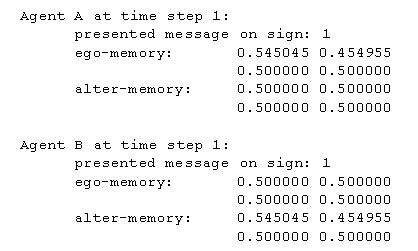2.22
As we can see, entry (1, 1) in A's ego-memory has increased, whereas entry (1, 2) has decreased. The same is true for B's alter-memory. Now it is B's turn. B has to react to A's activity 1. So, B calculates: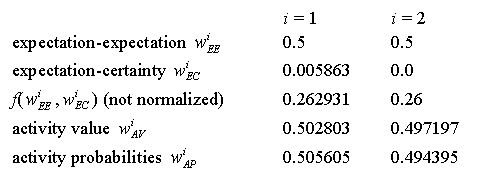2.23
We can observe that the expectation-certainty for activity i = 1 is greater than for i = 2 now, since B has already observed one time how A has reacted to activity 1. See the first row of B's alter-memory from which the expectation-certainty of activity 1 is calculated (0.00586 = fcertainty(0.545045, 0.454955)).

2.24
We can also see that the activity probability for activity 1 (0.505605) is larger than its activity value (0.502803). This is a result of scaling by g with exponent γ = 2 (quadratic selection). To give an example for how to calculate the activity probability for activity 1: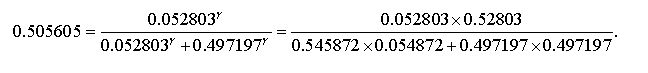(12)

2.25
B selects an activity randomly with probability of about 51% for activity 1 and probability of about 49% for activity 2. In our example Agent B selects activity 1. After storing the activity pair (1, 1) in B's ego-memory and A's alter-memory, the state of both agents is as follows: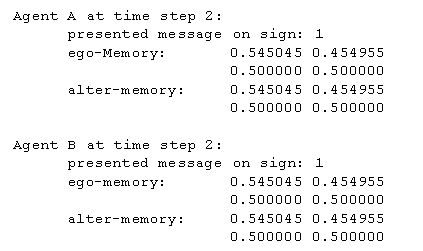2.26
Now it is A's turn. He has to react to B's activity 1. So, A calculates: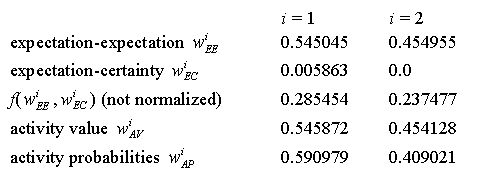2.27
We can see that A's expectation- expectation for activity 1 (0.545045) is larger than the expectation-expectation for activity 2 (0.454955). This means that A thinks that B expects activity 1 more likely than activity 2 to be performed by A. Now, A selects an activity randomly with a probability of about 59% for activity 1 and a probability of about 41% for activity 2. And so on...

###Results

3.1
We have performed a large number of simulation experiments in order to investigate the behavior of our model. There are three main results:

In the dyadic situation (two agents) order appears for a wide range of parameter settings.

1. We may say that "Luhmann has been right" that the process he describes leads to order in the dyadic situation. In a way this is a trivial result, as, e.g. also stated by Parsons, who clearly saw that the dynamics within sub-systems may generate a kind of ordered "coevolution". Our model, however, allows to study further, which factor possesses which kind of influence, as we shall show in Section 5. For a more detailed analysis of the dyadic situation see Kron and Dittrich (2002).
2. The appearance of order in the dyadic situation does not necessarily allow to infer that order appears in the multi-agent case. In Section 6 we show that the scalability of order formation depends critically (a) on how the agents calculate their expectation-expectation and (b) on the presence of a mechanism that allows information transmission between agents, in our case achieved by introducing observers.
3. Our model allows to demonstrate the transition from an actor-oriented perspective to a system level perspective. In Section 7 we demonstrate how we can derive an activity system from the microscopic interactions of agents. The communication system allows to describe a situation (state) independent from the underlying population of agents.

###How to Measure Social Order?

4.1
Before we can observe order formation in our model, we have to clarify: What does it mean for a system to be ordered? Here order is measured in the following ways:

1. Average number of different activities selected during a time interval
We measure the average number of different activities used by the agents during a time interval of a constant number of steps (here, 50 steps). The lower that number, the higher the order, because the larger is the contingency reduction. That is, for an observer order appears if there are few alternatives that come into consideration for the agents. This measurement, however, makes only sense if the number of different activities in that interval is much smaller than the length of the interval. (Data can be found in log-file: runName.msgstat)

2. Certainty of activity values - average certainty OAV
One elegant way to measure order is to apply the same function used to calculate certainty. In a way, in doing so we would take an actor-oriented perspective to measure order. The certainty function is simply applied to the activity values or activity probabilities of an agent, respectively. Thus the resulting values estimate how certain an agent is when he selects a message. A high value represents high certainty and thus high order. Formally we define:(13)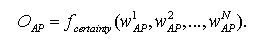(14)

In the following we will use OAV only, since it is very similar to OAP and because OAP taking into account has not lead to different conclusions. (OAV and OAP data can be found in log-file: runName.log)

3. Predictability of an activity - systems level order OP
Here we measure how predictable an activity of a randomly drawn agent Ego is, given the activity presented on the sign by another randomly drawn agent Alter. To measure the predictability we can use fcertainty again: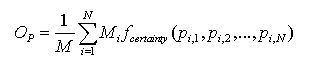(15)

where pi,j is the probability that a randomly drawn agent reacts with activity j to the displayed message i of Alter. Mi is the number of agents displaying message i. The matrix (pi,j) can be interpreted as the average behavior matrix of the whole population. In order to get an intuitive understanding of the systems level order imagine the following game: An external player has to predict the activity of the agents. At the outset the player can take a look at the internal state of every agent. Then, in each turn, an agent is chosen randomly, the activity number i on his sign is shown to the player. (Note that the agents are anonymous, so that the player does not know which agent possesses what kind of internal state). Then, again an agent is chosen randomly from the population and the player has to predict the reaction of that agent to the activity number i. For each correct prediction the player receives a point. The state of the agents is not altered during the game, so they are not allowed to learn during the game. The larger the systems level order OP is, the higher is the maximum average score that the player can achieve. For OP = 0 the player cannot perform better than just guessing randomly. For OP = 1 the player can predict the reaction correctly in each turn. Note that this measure makes sense only if the number of agents is large compared to the number of messages actively used. Sociologically we can interpret the value OP as a measure of integration. Integration with regard to the whole society, "social integration", is a very important term in sociological theory even if it is not definitely clear what it means, because the integration of society could be observed from different analytical perspectives (Münch 1997). The core of social integration consists of a situation of society where all of its particles are stably affiliated with each other and build up a unit which is marked-off outwards. So, in modern societies on the one hand we can differentiate between economical integration, the accentuation of exchange, free contracts, and the capitalistic progression of wealth; political integration, the accentuation of the importance of the exertion of political enforcement by means of national governance; cultural integration, the accentuation of compromise by discourse on the basis of mutually shared reason; and solidarity integration, the accentuation of the necessity of modern societies to generate free citizenships in terms of networks of solidarity. On the other hand we can observe the systemic integration (Schimank 1999). The accentuation here lies on the operational closure of the system. If a (activity) system is operationally closed it is in the position of being able to accept highest complexity of its environment, to pick up and to process this complexity in itself without to imperil its existence. We interpret the value OP as a measure of systemic integration in this sense. In all our simulation experiments a high value of OP has indicated a closure of the emerging activity system. This means that the better the agents are able to predict the activities of other agents as reactions to their own activity selections, the more the communication system appears to be operationally closed, that is certain activities follow certain activities.

###Behavior of the Basic Dyadic Model

5.1
In this section we analyze the properties of our basic model by systematic simulation experiments. In the basic model, only two agents are present interacting alternately. We investigate this "dyadic world" intensely, because it is the situation as described by Parsons and Luhmann. In the next section (Section 6) scalability is investigated, i.e., the number of agents is increased.

5.2
For the dyadic world we investigate the influence of various parameters, namely, (1) the number of possible activities N, (2) the influence of the selection method y (equal to the influence of randomness), and (3) the influence of the EE-EC weighting factor a. In order to show the average behavior of the model we have performed at least 20 independent runs for each parameter setting, only varying the random seed. Before looking at the average behavior we present a single run as example.

### Example of a Single Run

5.3
Figure 2 shows a typical complex simulation experiment of the dyadic situation. There are N = 64 potential activities. The agents are using expectation-expectation only (a = 0.0) and the selection method is something in between proportional and quadratic selection (γ = 1.5). The parameter setting is an example for a case where complex behavior appears.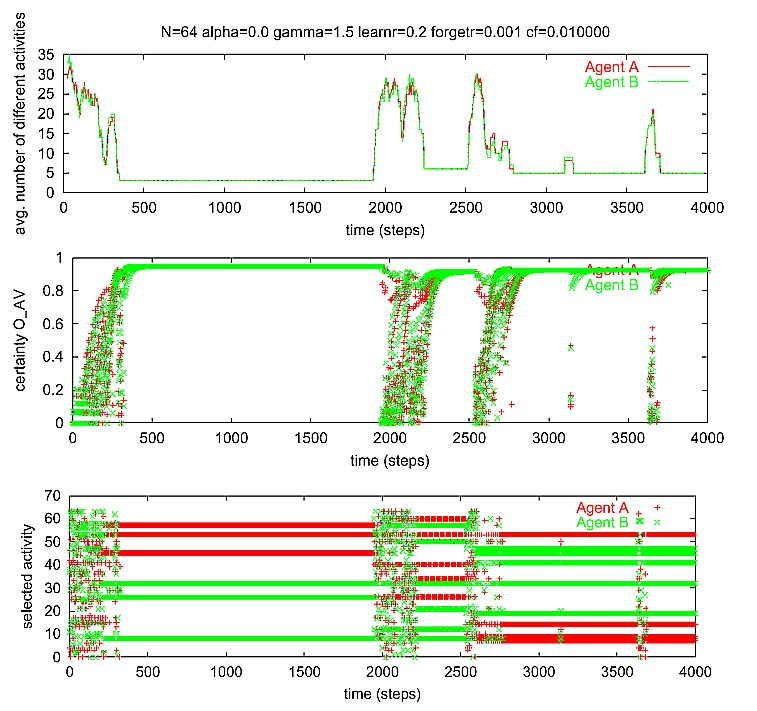Figure 2. A complex example of a single run of the dyadic situation. There are N = 64 potential activities. The agents are using expectation-expectation only (a = 0.0). The selection method is something in between proportional and quadratic selection (γ = 1.5). Learning rate rlearn = 0.2. Forgetting rate rforget = 0.001. cf = 0.010000.

5.4
We can see in Figure 2 that at the outset the average certainty of the agents is low. This means that they are not very sure about what to do, because their memories do not contain much information. Therefore, the calculated activity values are similar. An activity is selected more or less randomly, so that at the beginning many different activities are performed. Then, during learning, the number of different activities decreases quickly. So, in the so called transient phase (about step 0 - 400) the certainty increases, on average. In generation 400 a highly ordered state appears where the agents are sure what to do and only a few activities are used. We will refer to that state as an emergent activity system later (Section 7). Here, each agent uses four different activities, but Agent A acts different from Agent B. Both agents are sure what the other one expects. So the situation is quite stable.

5.5
Interestingly, at time step 1950 a heavy disturbance appears, which leads to a drop of the average certainty and a relatively complex, much more disordered phase (step 1950-2200). How could it happen that an activity system which is stable over a relatively long time breaks down after such a disturbance? We can only understand this system-breakdown if we analyze the agents:

5.6
Agent B initiated the disturbance by an unexpected activity, which confused the other agent (Agent A): This means that A was not able to calculate the expectation- expectation of B in that situation, since B suddenly used a completely unusual activity. Hence agent A reacts more or less randomly. This nearly random reaction confuses agent B in turn. The mutual confusion may be amplified, as is the case in this example, by further reactions and result in a heavy disturbance, which later gives way to a new relatively stable state. This new stable state consists of an activity pattern with five different activities used by each agent.

5.7
In this example we can also see that an activity pattern (or system) can be stable against small disturbances which appear, for instance, at steps 3200 and 3700. Note: Given that agents sometimes act randomly, we cannot expect (or deduce) that periods of stability and periods of instability exist for chance reasons. A small (structural) change of the model may lead to a new model where the "periods of stability" are asymptotically stable with large basins of attraction. Under those conditions disturbances caused by occasional random activities would not lead to a situation of instability.

#### Influence of the Number of Activities N

5.8
According to Luhmann the number of possible activities N has a strong influence on the genesis of social order, since contingency reduction increases with increasing number of activities.

5.9
In order to investigate the influence of the total number of allowed activities N, we performed simulations with learning rate rlearn = 0.2, forgetting rate rforget = 0.001, and different EE-EC factors a = 0.0, 0.5, 1.0.

5.10
Figure 3 shows how the average number of different messages in an interval of 50 times steps depends on the total number of possible activities N (for proportional selection, y = 1).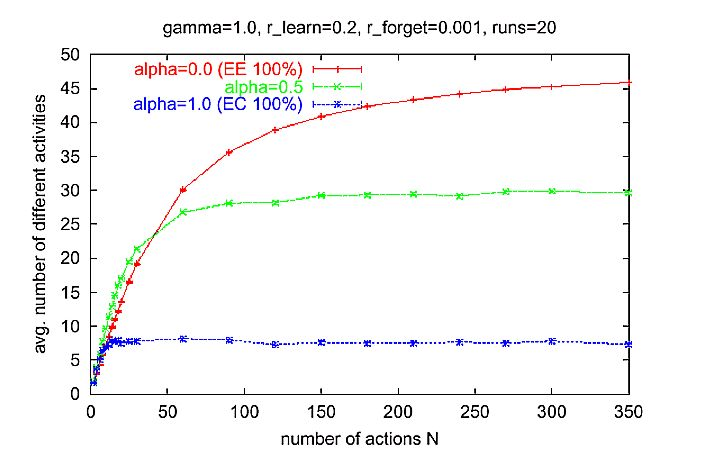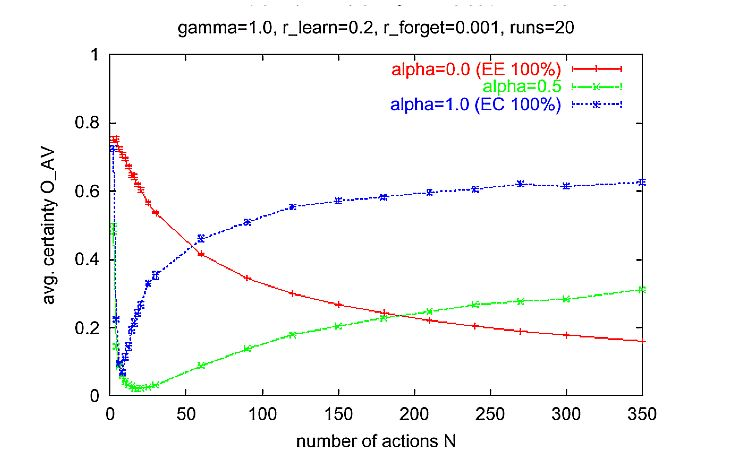Figure 3. Average number of different activities in an interval of 50 time steps for different N. Measurement started at time 500 after the transient phase at the beginning. Simulation time: 1000 time steps for each run. Parameter setting: normal learning rate rlearn = 0.2, low forgetting rate rforget = 0.001, proportional selection y = 1.

5.11
Let us take a look at the curve for a = 0.5: We can see that activities are not chosen totally at random and that there must be a certain order. The green curve in the upper diagram of Figure 3 can be interpreted as follows: If we look at a randomly chosen interval of 50 time steps (t > 500) and count the number of different activities appearing in that interval, we will observe, on average, about 29 different messages for large N (e.g., N = 300). This is much less than the number of different messages one would observe, if agents chose their activities randomly out of {1, 2, ..., N}.

5.12
If we decrease the influence of randomness by increasing y, this effect becomes more pronounced. Figure 4 shows the same as Figure 3, but with y = 1.5. We can see that, as expected, the number of different activities used by the agents is much smaller than for y = 1.0. The degree of order is quite high and does not decrease for an increasing number of possible activities. Thus, it is reasonably safe to conclude that order appears for an arbitrarily large number of possible activities, provided y is chosen sufficiently high.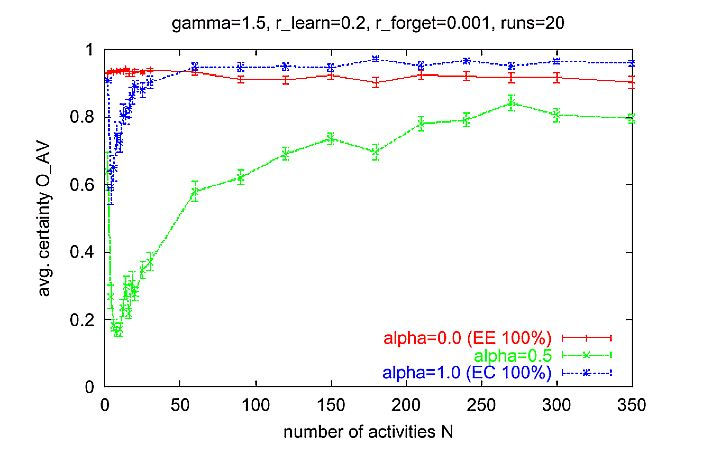Figure 4. Same as in Figure 3, but with a lower influence of randomness (y = 1.5).

### Influence of the Selection Method y and EE-EC Factor a

5.13
As said before, the activity selection of an agent depends on two factors, namely, expectation- expectation (EE) and expectation-certainty (EC). Both factors are lumped together by a weighted sum, with a the weight for EC (Eq. (2)) and (1-a) the weight for EE. Roughly speaking, the larger a, the more an agent tries to select an activity such that the future becomes predictable. A small a means that an agent tries to meet the expectation- expectation of the other agent. In Figure 5 we can see how the average number of different messages in an interval of 50 steps and the average certainty OAV depend on the relation of EE to EC a.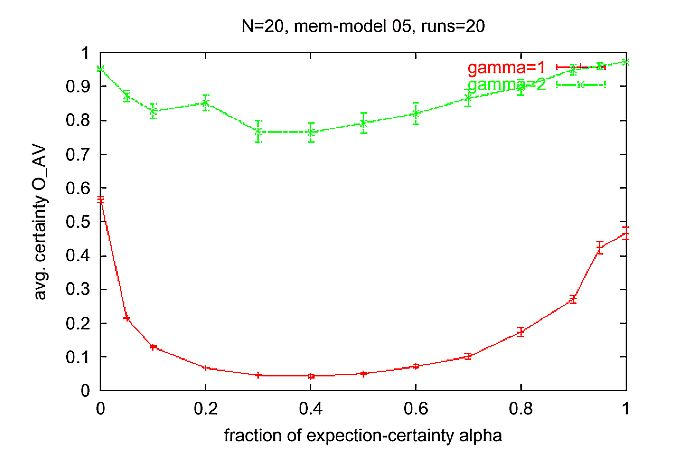Figure 5. Average number of different activities in an interval of 50 time steps for different a. Measurement started at time step 500, such that the transient phase at the beginning is not considered. Simulation time 1000 time steps for each run. Parameter setting: normal learning rate rlearn = 0.2, low forgetting rate rforget = 0.001, number of activities N = 20.

5.14
This leads to an interesting result: If agents take into account the expectation-expectation (EE) only, or if they take into account the expectation-certainty (EC) only, a relatively high average certainty OAV results. If a mixture of EE and EC is used, e.g., a = 0.5, then the average certainty OAV is much lower. It is interesting to note that for a = 0 (EE only) the average expectation certainty OAV is high (about 0.58), even if the average number of different activities used is large (about 15 activities).

5.15
In our model the selection method depends on a parameter γ∈ [0,∞]. For y = 0, agents choose their activities totally randomly from the set {1,2,...,N} of possible activities (each activity with same probability). In Figure 3 we showed experiments with y = 1, in which the probability that an activity i is chosen is proportional to its activity value wiAV.

5.16
Figure 6 demonstrates how the behavior of our model depends on y. We can see that for increasing y (increasing determinism of activity selection) the influence of a on OAV and on the average number of different activities used is reduced. But looking at Figure 6 we can see also that the influence of a is high only for the transition phase from disordered to ordered behavior (0.5 < γ < 2). We will see that in the multi-agent case a will become much more important.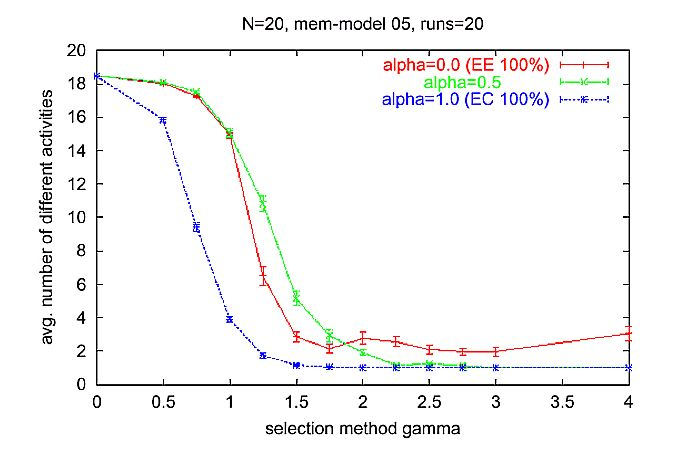Figure 6. Average number of different messages (activities) in an interval of 50 time steps for different y. Measurement started at time step 500 after the transient phase at the beginning of runs. Simulation time 1000 time steps for each run. Parameter setting: normal learning rate rlearn = 0.2, low forgetting rate rforget = 0.001, number of activities N = 20.

5.17
If we look at the correlation between a and y for y < 1.2 we can observe an interesting phenomenon: Just trying to meet the expectations of the other leads to a better predictability of the future than choosing an activity according to an estimate of future's predictability based on the own experience (memory). This phenomenon, however, is not general, rather it appears for specific parameter settings (in our case γ < 1.2, see Figure 6) and seems to be amplified especially in the multi-agent scenarios (see Section 6).

###Scaling Up - The Behavior of a Population of Many Agents

6.1
In this section we will investigate the scalability of our model. We will look at the behavior of many agents interacting based on the same mechanism as in the previous section. Note that Luhmann has described the situation of double contingency as an interaction of two entities. But there is no discussion in the literature how this might scale up. Luhmann's answer to this problem is that systems would "emerge" from the outset of the situation of double contingency and would sustain themselves through self-organization (see also Luksha 2001). Thus, an investigation of scalability would not be necessary because social systems and psychic systems would be strictly separated by their operations (communication vs. thinking). Exactly at this point a more detailed explanation of the emergence is missing (Esser 2000, pp. 1- 29): Luhmann does not explain if and how the genesis of social systems is possible in the case of multi contingency, although multi contingency is more "empirically realistic" than double contingency. And, of course, multi contingency is a non-linear phenomenon and cannot just be thought of as a summation of double contingency situations (assuming linearity).

6.2
As we will see in the following section, scalability in the terms of increasing numbers of agents decreases the probability for the emergence of order dramatically. In order to arrive at an ordered behavior we have to choose our parameters much more carefully than for the dyadic situation.

The aim of the following investigation is to identify those parameters and mechanisms that are important for the formation of order under the condition of increased numbers of agents.

### Using the Ego-Memory to Calculate the Expectation-Expectation

6.3
In order to simulate a population of many agents we have to change the algorithm for interaction slightly:

1. Randomly choose an agent from the population and call it Ego.
2. Randomly choose another agent and call it Alter.
3. Let Ego observe Alter's displayed message a (equal to Alter's last activity).
Let Ego react to Alter's message. (Note that only Ego acts, but not Alter.)
4. Ego stores its reaction b in its Ego-memory.
Formally, Ego does: Mego := memorize(Mego, a, b).
5. Alter stores Ego's reaction b in Alter's Alter-memory.
Formally, Alter does: Malter := memorize(Malter, a, b).

6.4
Figure 7 shows that for a small number of agents, systems level order is present. In that situation a single agent behaves deterministically but usually it behaves differently than other agents. Thus there is order but not a common activity pattern. From the perspective of an agent the behavior of the other agents appears to be disordered, because it is not able to identify individuals. As a result, low systems level order OP can be observed if we increase the number of agents.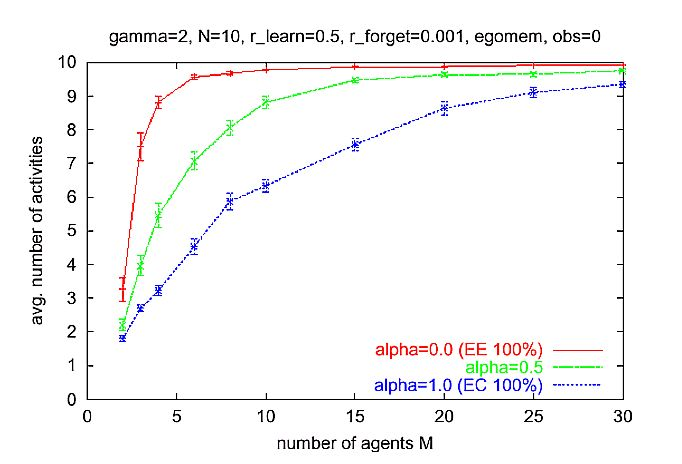Figure 7. Average behavior of multi-agent simulations where the Ego-memory is used for calculation of the expectation-expectation, as in the basic dyadic scenario. Parameters: N = 10 activities, y = 2 (quadratic selection), rlearn = 0.5, rforget = 0.001, cf = 0.01. Multi agent scenario as described in Section 6.1

6.5
Why are agents in large populations not able to predict correctly the expectations of others? The reason is that an agent (Ego) calculates the expectation-expectation based on its Ego-memory. Thus Ego uses its own past behavior to predict what the other agent (Alter) expects from it. This works fine in a dyadic situation, because the other agent has observed the past behavior of Ego. But in a larger, randomly interacting, population it is unlikely that Alter has met Ego before and thus the expectations of Alter are (mostly) independent of Ego's past behavior. Hence we conclude: For scalable prediction ability Ego must use more information than solely the memory entries of its own past behavior.

### Using the Alter-Memory to Calculate the Expectation-Expectation

6.6
Now we implement a small but important change in our model. In the basic model an agent has calculated the expectation-expectation by using its Ego-memory. That means that Ego expects from Alter that Alter expects from Ego that Ego acts similarly to how Ego acted in the past.

6.7
But now an agent uses its Alter- memory instead. That means that Ego expects from Alter that Alter expects from Ego that Ego acts similarly to how other agents acted when encountered by Ego. So Ego is not using its experience of own activities to generate the expectation-expectation. Rather, it uses the average behavior of others reacting to his own activities.

6.8
The simulation results are shown in Figure 8. First note that the curves for a = 1 (EC only) are the same as in Figure 7, since for a = 1 only the expectation-certainty is used for activity selection and we have only changed the way how the expectation-expectation is calculated, which does, however, not influence the EC calculation.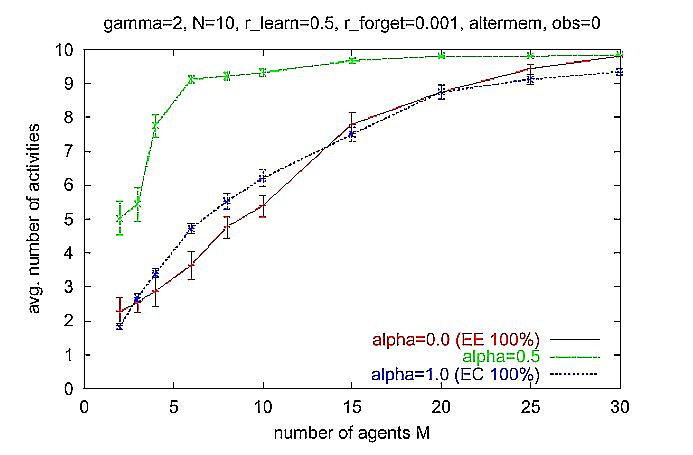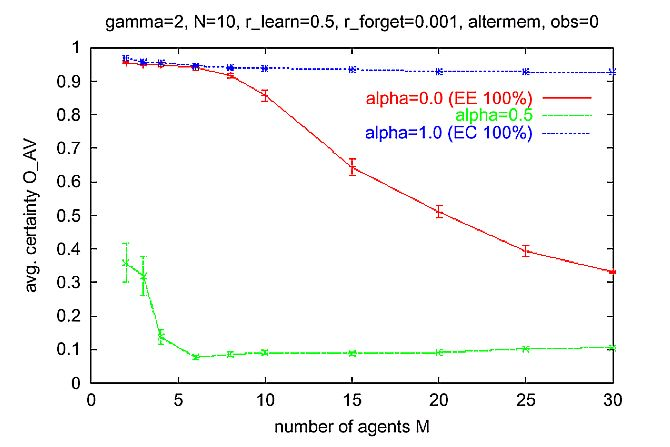Figure 8. Same as Figure 7, but Alter-memory is used to calculate expectation-expectation (EE). Multi-agent scenario as described in Section 6.1.

6.9
Looking at the red curves, we can see that for a = 0.0 (EE only) the systems level order OP is much higher than in the previous case (Section 6.3). But, as in the previous case, the systems level order is not scalable and systems level order OP decreases with an increasing number of agents M. Only for a moderate number of agents (M < 10) we can observe nearly maximal systems level order (OP close to 1). Thus we can randomly chose two agents from the population, and can predict exactly how one agent would react to the other, without knowing the internal state of the acting agent.

6.10
The important difference to the previous case is that now every agent acts in the same way (as we will see later). So there are shared common activity patterns, which we will regard as an activity network later on. But, as noted before, this shared behavior can only be "learned" in small populations (M < 10).

6.11
Why is Ego still unable to predict correctly the expectations of others in large populations? The reason is that Ego observes Alter's behavior only as a reaction to its own (Ego's) behavior. For instance, if Alter shows a sign (activity) that Ego has never used, Ego cannot predict what Alter expects, because Ego has never encountered an agent that has reacted to that activity.

6.12
We can conclude: For scalability it is not enough that Ego uses its memory entries of the behavior of other agents that react to its (Ego's) activities. It might be interesting to note that, although the systems level order is higher and the average number of activities is smaller than in the previous case of using the Ego- memory, the certainty of a single agent OAV is lower (for a = 0, and especially for M > 10). It seems that if agents consider only EE, they are more confused despite a higher systems level order. This does not mean, however, that the consideration of EC automatically leads to higher systems level order or certainty. If we look at the green curves showing a mixture of EE and EC (a = 0.5), we observe an extremely low systems level order OP, a low average certainty OAV, and a high number of different activities. In sum, the whole system is less ordered.

6.13
In a further step we extend the model by allowing an agent to observe the interaction of others. For this purpose the algorithm is varied.

In each simulation step, do:
1. Randomly choose an agent and call it Ego.
2. Randomly choose another agent and call it Alter.
3. Let Ego observe Alter's displayed message a (equal to Alter's last activity).
Let Ego reacts to Alter's message. (Note that only Ego acts, but not Alter.)
4. Ego stores its reaction b in its Ego-memory.
Formally, Ego does: Mego := memorize(Mego, a, b).
5. Alter stores Ego's reaction b in Alter's Alter-memory.
Formally, Alter does: Malter := memorize(Malter, a, b).
6. Choose n agents randomly and call them observers.
7. Each observer stores Ego's reaction in its Alter-memory.
Formally, each observer performs Malter := memorize(Malter, a, b) where a is the message displayed by Alter and b is Ego's reaction.

6.14
Note that in every simulation step it is determined anew, who is Ego, Alter, or an observer. So, the same agent can be Ego in one step and an observer in the next step. Figure 9 shows that, opposite to the results shown before, systems level order OP corresponds to the average certainty OAV of single agents.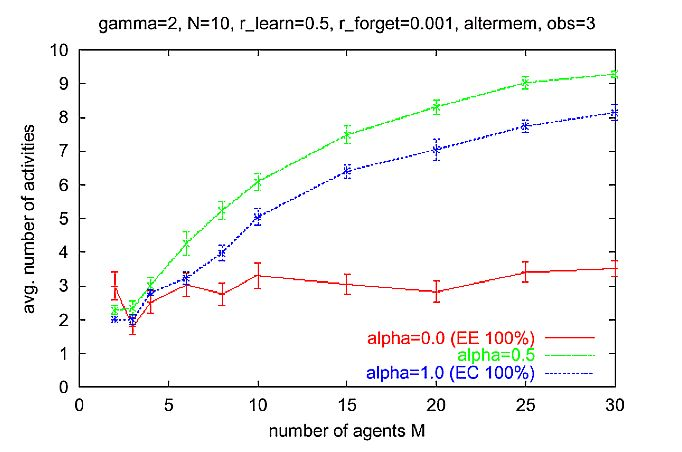Figure 9. Same as Figure 8, but with n = 3 observers. For a = 0 (EE only), systems level order is scalable. As in Figure 8, the Alter-memory used to calculate the expectation-expectation. Multi-agent scenario as described in Section 6.13.

6.15
But only for a = 0 (EE only) the systems level order is high and is scalable; i.e., in all simulation experiments with a = 0 (EE only) an activity pattern appeared with maximum systems level order and maximum certainty of agents. One may say that the activity system is completely integrated and closed (neglecting small random fluctuation due to the constant cf). What is more: This phenomenon is scalable! With increasing number of agents order still appears, in other words the appearance of order is independent of the number of agents. But as said before this is true only for a = 0 (EE only).

6.16
If Luhmann says that expectation- expectation is necessary to generate social order then we can add: if we do not consider further extensions, such as trust or social networks, in our model the scalability of social order is just possible under the exclusive consideration of expectation-expectation (a = 0 , EE only). If expectation-certainty is considered, systems level order (systemic integration) breaks down with increasing number of agents M for a = 1 (EC only) as well as for a = 0.5. Thus, scalability is achieved if (1) Ego uses its alter-memory (which stores interactions among other agents Ego has observed) to calculate the expectation-expectation, (2) Ego uses expectation-expectation only for activity selection, and (3) there is at least a certain (minimum) number of observers who observe activities and learn from these observations.

###Emergence of Activity Systems - A Systems Level View

7.1
We have started this paper from the microscopic level by specifying the agents and how they act. Although one can say that Luhmann has also described the situation of double contingency in an actor-oriented way, the main body of his theory uses a systemic view and does not require any notion of an actor. In this section we shall therefore also move from the actor-level description to a systems level description. That is, we shall describe the emerging activity systems in our simulation experiments as networks (Fuchs 2001) or graphs. This may also help to understand what is meant by a systemic view of a society.

### Definition of Activity Systems

7.2
Recall that we assume a population of M agents and that each agent can select from N different possible activities {1, 2, ..., N}. Execution of an activity is equivalent to displaying a sign with the activity number on it (Figure 1). Each agent displays only one sign with a number at any time. For activity selection an agent looks at the sign of a randomly chosen agent and reacts to the presented number.

7.3
We define the activity graph as a directed graph (V, E), where the vertices (nodes) are possible activities: V = {1, 2, ..., N}. Two nodes v1, v2V are connected by an edge (v1, v2) ∈ E, if and only if there is an agent in the population that would react to v1 by activity v2. For each edge we can define a weight w(v1, v2) as the probability that a randomly chosen agent from the population reacts with v2 when seeing activity v1. (Note that v1 need not to be shown by any agent in the population.)

7.4
For analysis and visualization it is convenient to look at a reduced graph: In an activity graph with edge threshold r we keep only those edges whose weights are larger than a threshold r . For our model this is appropriate, since agents can react with a low probability with any activity (if cf > 0 and y < ∞, see also Section 9.1). Additionally we can remove nodes that do not have any incoming edges.

7.5
In terms of general systems theory an activity graph is a system. But from the point of view of Luhmann's systems theory, we may not call every activity graph a system. In Luhmann's theory it is important that there are inner elements belonging to the system and that there are outer elements belonging to its environment. The system-environment distinction is the precondition for observing systems.

7.6
How should we define an activity system more formally? A first attempt might read:

An activity system consists of a set of activity symbols (subset of {1, 2, ..., N}) where activity symbols within that set mainly produce activity symbols within that set, and every activity symbol in that set is produced by activity symbols of that set. This can be expressed more formally, e.g.: The set O,O ⊆ {1,2,...N}, is called activity system, if and only if (1) for all v1O and v2O,w(v1, v2) ≤ r, (property of closure), and (2) for all v2O there exists v1O such that w(v1, v2) > r (property of self-maintenance).

7.7
The threshold is one way to formalize the fuzzy term "mainly" of the previous informal definition. With this definition an activity system is equivalent to a (chemical) organization as defined by Speroni et al (2000) following Fontana and Buss (1996). This equivalence allows us to view activity systems as artificial chemistries (Dittrich et al 2001), which may open a path for a promising and powerful theoretical treatment.

### Examples of Emergent Activity Systems

7.8
Figure 10 shows a typical activity graph that has emerged in a simulation experiment with M = 20 agents, N = 64 possible activities, and no observers.Figure 10. Typical activity system that has emerged in a simulation experiment with 20 agents, 64 possible activities, and no observers. A node represents an activity. All nodes without any incoming edges are removed, except for one (upper right corner of the diagram), which should illustrate how the removed nodes are connected to the "inner" active network. Parameters: y = 2, N = 64, a = 1:0; rlearn = 0.2, rforget = 0.001, M = 20. No observers. Ego-memory used for EE calculation. Threshold = 0.01. The corresponding single run is shown in the Appendix, Figure 13.

7.9
In this particular case, the agents are using just 15 out of 64 available activities. Using the definition above, we can call the set of 15 activities the elements of an activity system, which can be distinguished from the remaining elements (activities). There is a transition from every node to every other node within the system, but there is no transition leading to outer elements, except for those transitions which occur with very low probability (smaller than 0.01) and which are excluded by the threshold r = 0.01. These interferences (perturbations) cannot influence the system in a deterministic manner. An activity outside the system (exemplified by the node in the upper right corner) would lead to an activity within the system. So, there is a certain order, which is reflected also by our systems level order measure equal to OP = 0.39 in the situation shown in Figure 10.

7.10
In Section 6.3 we have shown that for the parameter setting used in Figure 10, order disappears if the number of agents is increased. Why is the system not scalable? This becomes clear if we look at agents and how they act. As also shown in Section 6.3 the average certainty of activity values OAV is maximal. This means that every agent is 100% sure of what to do. But every agent is doing something different (by chance, though, some agents are doing the same). There is no common "language" or common activity pattern. In fact, every agent selects only one specific activity - all the time the same - independently of the activity it has encountered. This explains why systems level order is present only in a small population of agents and why there is a transition from each node of the activity system to every other node within the system.

7.11
Figure 11 shows an example of maximal systems level order OP . The example is taken from an experiment with M = 10 agents and N = 10 possible activities with agents only using expectation-expectation based on their Alter-memory. (Similar networks appear for "scalable" parameter settings where observers are included). The agents in the population use only three activities, namely O = {1, 5, 6}. Within that activity system transitions are practically deterministic: Each agent acts in the same way, which is an important difference to the previous example. There is a common activity pattern that is shared among all agents. The structure of the remaining network is a reminiscence of the process in the past during which the activity system O emerged (see Figure 14 in the Appendix).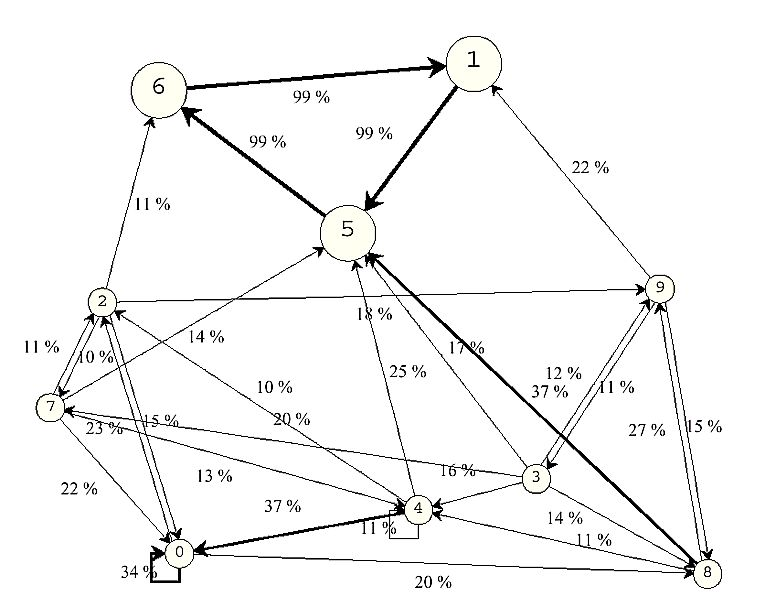Figure 11. Example of an activity system that has emerged in a simulation experiment with 10 agents, 10 possible activities, Alter-memory used for EE calculation, and no observers. A node represents an activity. The sub-set of nodes {1, 5, 6} can be interpreted as an autopoietic (sub-)system. Parameters: y = 2, N = 10, a= 0.0, rlearn = 0.2, rforget = 0.001, M = 10. No observers. Alter- memory used for EE calculation. Edges with weights smaller than r = 10% have been omitted for clarity. The corresponding single run is shown in the Appendix

### Is there Autopoiesis?

7.12
The results of our simulation experiments show that activity systems have emerged. But are they autopoietic, like Luhmann stated social systems would be? Per definitionem (by Luhmann), they are not, since social systems consist of communication and not of activities. But the example of an emerging activity system in our multi-agent world in Figure 11 shows that activities 1, 5, and 6 are completely interlinked. Even (small) disturbance by other activities do not produce "resonances" the system is not able to cope with. Usually, the system equilibrates itself quickly. In concordance with Luhmann the activity system is reproduced by an ongoing development through the production of system elements by elements of the system (Luhmann 1988, p. 71). This is, what autopoiesis means for Luhmann: Elements are elements just for systems which use these elements as unity, and the elements are a unity just by the means of the system (Luhmann 1984, p. 43). No individual, person, human being or psychical system, "nothing non-social" is directly and indispensable involved in the reproduction of the communication system, if we only take the macroscopic observer perspective. If we look at the network in Figure 11 we cannot see the acting agents "behind" this network. The network is independent from its agents, it operates autonomously (this does not mean: autarchic!). From the vantage point of agents, the network could be a fiction that sustains itself, since the agents deal with the network as if it would be real and so it is real as a consequence. In other words, we may interpret the network as a fiction of its actors, generated and sustained as a self-fulfilling-prophecy (Schimank 1988).

###Conclusion

8.1
In this paper we have demonstrated how a component of a social theory can be formally modeled and analyzed by simulation in order to reveal its critical determinants. Concretely we have modeled the situation of double contingency as a fundamental problem in the context of the formation of social order.

8.2
We have investigated a number of factors, such as the memory capacity of agents and the activity selection method. In summary, we can say that the mechanisms proposed by Luhmann and others lead to order in the dyadic case. Taken together, the most important factor in the dyadic situation is the activity selection mechanism, or more precisely, the influence of randomness, which is closely related to how well agents are able to perceive their world (including other agents). The missing description of the activity selection mechanism is an important deficit in Luhmann's and Parsons' theory (Esser 2001, pp. 33-78), since it is so fundamental for an explanatory sociology (Esser 1993). Luhmann has not specified a rule according to which an entity selects an activity among a set of potential alternatives. Therefore, one cannot explain why and under which specific parameter settings systems appear.

8.3
Our thesis is that Luhmann can dispense of this rule if there are systems, but that he cannot dispense of it for the purpose of explaining the genesis of a system. The reason why Luhmann did not (want to) consider the activity selection mechanism is that - in his view - social systems evolve independently of certain actor qualities. Our simulation experiments show that without the consideration of these qualities an explanation of the genesis of autopoietic communication systems is not possible or would become trivial. The capacities to perceive, memorize, generalize, and to make predictions are important properties of social actors. This kind of "cognitive" capacities should also be present in a computational agent modeling a social actor.

In Section 6 we have shown that the scalability of order formation depends critically (a) on how agents calculate their expectation-expectations and (b) on the presence of a mechanism for information transmission between agents, in our case achieved by introducing observers. The resulting behavior is similar to learned imitation (Ikegami and Taiji 1999; Conte and Paolucci 2001). We have found that for scalable systems level order, (a) Ego must not use (solely) its memories of his own past behavior to predict what Alter expects from it, (b) it is not sufficient that Ego uses its memories of the behavior of other agents that react to its (Ego's) activities. Scalable systems level order appeared if (a) the agents use only expectation-expectation for activity selection, and (b) if the expectation-expectation is generated from observations of the interaction of other agents. If agents included expectation-certainty into their decision process, scalable systems level order has not been observed for any parameter setting investigated in this paper. We think, however, that this should not be taken as a general result yet, before we are able to explain this phenomenon more theoretically and before we have performed further simulation studies with different memory and certainty models. Finally, a third important result is that our model allows to demonstrate the transition from a more actor oriented view to a systems level view. Therefore it helps to understand the so called "micro-macro-link", a fundamental problem of sociology, which is concerned with the question, how an over-individual aggregation, e.g., communication system, emerges from interaction of many actors. For a "complete" explanation, the logic of aggregation has to be examined in detail. One would have to pin down the coherence of transformation rules, transformation conditions, and the "individually" explained individual effects (Esser 2000, pp. 18-29). In further studies this may lead to a more precise notion of closure, self-reference, self-production, and autopoiesis of communication systems. An important step in our future research will be the introduction of social relationships, i.e., the introduction of a topology, a spatial differentiation. In our model each agent interacts with every other agent with the same probability. In the real world, however, this probability depends on geographical conditions and the social network among actors. Obviously this social network plays an important role in the formation and persistence of social order. A dynamic social network can be easily introduced by a process where a "successful" interaction amplifies a social connection (Skyrms and Pemantle 2000). It would be extremely interesting to investigate the resulting coevolution of the social and the activity network.

###Acknowledgements

We are grateful to Gudrun Hilles, Christian Lasarczyk, Uwe Schimank, Andre Skusa, and to the anonymous referees. The project is funded by the German Research Foundation (DFG), grant Ba 1042/7-2 and Schi 553/1-2. PD is also funded by the German Federal Ministry of Education and Research (BMBF, grant 0312704A). A JAVA version of the simulation model called "LuSi" implemented by first of all Christian Larsarczyk, and Oliver Flasch and Frank Rosseutscher is available under: <http://www.fernuni- hagen.de/SOZ/SOZ2/Projekte/Sozionik/english>.

###AppendixThe Appendix contains further details about the model and about the simulation software that was used for the experiments described in the paper. To display the appendix, which is in , in Portable Document Format (PDF), you will need a copy of the Adobe Acrobat reader, available free.

###Notes

1 In an earlier version, Parsons' (1951) solution for the problem of double contingency had a much more economical bias. See also Mnch (1986).

2 The term "entity" denotes what Luhmann (1988) called "Ego" and "Alter" in Chapter 3 (p. 148), and Parsons called "actor".

3 One of Luhmann's basic assumptions is that both actors are interested in solving this problem. Luhmann (1984, p. 160): "No social system can get off the ground, if the one who begins with communication, cannot know or would not be interested in whether his partner reacts positively or negatively." But the question remains: Where does the motivation (interest) come from? According to Luhmann, an answer should not consider actor characteristics (like intentions) as starting point for system theory. We think that Luhmann falls back to his earlier anthropological position (see Schimank (1988, p. 629; 1992, p. 182)) and assumes a basic necessity of "expectation-certainty", that is, that Alter and Ego want to know what is going on in this situation. A fundamental uncertainty still remains and takes further effect in the emerged systems as an autocatalytic factor. See also the approach to formulate "double contingency" from the perspective of a communication network as provided by Leydesdorff (1993, pp. 58f.)

4 For new simulation experiments about the genesis of symbolic generalized media, see Papendick / Wellner (2002).

5 We prefer to use the term "activity" because Luhmann's concept of communication is much more complex than the communication processes among the agents in our model; see also Hornung (2001). We cannot use the term "action" as understood by Parsons, because our agents do not show a meaningfully motivated behavior that is oriented toward other agents according to certain goals, means, and a symbolic reference framework rooted in the situation. Therefore, our agents decide to do something we call "activity" - no more and no less. For Luhmann every communication consists of a selection triple based on the three distinctions (1) information, (2) transmission (German: Mitteilung), and (3) understanding (see Luhmann (1984, Chapter 4)). Our agents do not communicate in that sense, but we could take their interaction as an abstract model of communication dispensing from the distinction between message, information and meaning. Since it just represents the transmission of information, it is not a accurate model for Luhmann's communication concept. In this contribution, however, we are just interested in the process of order formation and have removed many details for the sake of simplicity.

6 Storing an event (a, b) in memory M is achieved by calling M':= memorize(M, a, b). M' is the new memory (or the new state of the memory), which is created by inserting (a, b) into M.

7 With increasing learning rate, the number of different messages, used by the agents, decreases (see Section 9.2 in the Appendix). Qualitatively, the relative behavior of the model is independent of the choice of the learning rate (above 0.2).

8 Alternatively we can measure the number of different activity- reaction pairs (a, b) occurring in a time interval.

9 Another important meaning of systemic integration in sociology is the integration of different social systems within society.

10 Note that in our simulation there is always a small chance for every activity to be selected.

11 "More or less randomly" means that there are some very small memory traces left. Thus the reaction is not fully dictated by chance. Note also that when we add expectation-certainty the situation will be different.

12 Recall that high systems level order means that just by observing the interactions among (non-learning) agents, it is possible to predict, how an agent in the population will react, without knowing his internal state nor his identity.

13 This non-linearity is not a general phenomenon, because it does not appear if using a different memory type like the linear degenerating memory (type 04), see Appendix Section 9.4.

14 Activity symbols are equivalent to an activity.

15 Note that we define "activity system" operationally for the purposes of our discussion here. The definition should show what can be interpreted as an activity system in our model. And the formal form should make that as clear as possible. It is, however, not a general definition of "activity system".

16 Here, "entity" refers to Ego and Alter in Luhmann's (1984) explanation of the situation of double contingency. Note that an "entity" needs not to be a human person.

17 In terms of Hempel and Oppenheim (1948).

###References

AXELROD, R. (1984). The Evolution of Cooperation. New York: Basic Books.

CONTE, R. / M. PAOLUCCI (2001). Intelligent social learning. In: Journal of Artificial Societies and Social Simulation 4 (1): pp. U61-U82.

DITTRICH, P., J. Ziegler, and W. Banzhaf (2001). Artificial chemistries - a review. Artificial Life 7(3), 225-275.

DURKHEIM, E. (1893). De la division du travail social. Paris (1968).

ESSER, H. (1993). Soziologie. Allgemeine Grundlagen. Frankfurt/Main: Campus.

ESSER, H. (2000). Soziologie. Spezielle Grundlagen. Band 2: Die Konstruktion der Gesellschaft. Frankfurt/Main: Campus.

ESSER, H. (2001). Soziologie. Spezielle Grundlagen. Band 6: Sinn und Kultur. Frankfurt/Main: Campus. FONTANA, W. / L. W. BUSS (1996). The Barrier of Objects: From Dynamical Systems to Bounded Organization. In: J. Casti / A. Karlqvist (Eds.), Boundaries and Barriers, Redwood City, MA. Addison- Wesley: pp. 56-116.

FUCHS, S. (2001). Networks. In: Soziale Systeme 7 (1): pp. 125-155.

HEMPEL, C. G. / P. OPPENHEIM (1948). Studies in the logic of explanation. In: Philosophy of Science 15: pp. 135-175.

HOBBES, T. (1651). Leviathan. In: W. Molesworth (Ed.), Collected English Works of Thomas Hobbes, No 3, (1966). Aalen.

HORNUNG, B. R. (2001). Structural coupling and concepts of data and information exchange: Integrating Luhmann into Information Science. In: Journal of Sociocybernetics 2 (2): pp. 13-26.

IKEGAMI, T. / M. TAIJI (1999, September 13-17). Imitation and Cooperation in Coupled Dynamical Recognizers. In D. Floreano / J.-D. Nicoud / F. Mondada (Eds.), Proceedings of the 5th European Conference on Advances in Artificial Life (ECAL-99), Volume 1674 of LNAI, Berlin. Springer: pp. 545-554.

LEPPERHOFF, N. (2000). Dreamscape: Simulation der Entstehung von Normen im Naturzustand mittels eines computerbasierten Modells des Rational-Choice-Ansatzes. In: Zeitschrift fr Soziologie 29 (6): pp. 463- 484.

LEYDESDORFF, L. (1993). "Structure" / "Action" Contingencies and the Model of Parallel Processing. In: Journal for the Theory of Social Behaviour, 23: pp. 47-77.

LOMBORG, B. (1996). Nucleus and shield: The Evolution of Social Structure in the Iterated Prisoner's Dilemma. In: American Sociological Review 61 (2): pp. 278-307.

LUHMANN, N. (1984). Soziale Systeme. Grundri einer allgemeinen Theorie. Frankfurt a.M.: Suhrkamp.

LUHMANN, N. (1988). Die Wirtschaft der Gesellschaft. Frankfurt/Main: Suhrkamp.

LUKSHA, P. O. (2001). Society as a Self-Reproducing System. In: Journal of Sociocybernetics 2 (2): pp. 13-26.

MNCH, R. (1986). The American Creed in Sociological Theory: Exchange, Negotiated Order, Accommodated Individualism and Contingency. In: Sociological Theory 4: pp. 41-60.

MÜNCH, R. (1997). Elemente einer Theorie der Integration moderner Gesellschaften. In: W. Heitmeyer (Ed.), Was h‰lt die Gesellschaft zusammen? Bundesrepublik Deutschland. Auf dem Weg von der Konsens- zur Konfliktgesellschaft, Band 2, Frankfurt/Main. Suhrkamp: pp. 66- 109.

PAPENDICK, S. / J. Wellner (2002). Symbolemergenz und Strukturdifferenzierung. In T. Kron (Ed.), Luhman modelliert. Sozionische Ans‰tze zur Simulation von Kommunikationssystemen, Opladen. Leske + Budrich: pp. 175-208.

PARSONS, T. (1937). The Structure of Social Action. New York: Free Press.

PARSONS, T. (1951). The Social System. New York: Free Press.

PARSONS, T. (1968). Interaction. In: D. L. Sills (Ed.), International Encyclopedia of the Social Sciences, Volume 7, London, New York: pp. 429-441.

PARSONS, T. (1971). The System of Modern Society. Englewood Cliffs.

SCHIMANK, U. (1988). Gesellschaftliche Teilsysteme als Akteurfiktionen. In: Kölner Zeitschrift fr Soziologie und Sozialpsychologie 4: pp. 619-639. SCHIMANK, U. (1992). Erwartungssicherheit und Zielverfolgung. Sozialität zwischen Prisoner's Dilemma und Battle of the Sexes. In: Soziale Welt 2: pp. 182-200.

SCHIMANK, U. (1999). Funktionale Differenzierung und Systemintegration der modernen Gesellschaft. In J. Friedrichs / W. Jagodzinski (Eds.), Soziale Integration, Opladen, Wies-baden. Westdeutscher: pp. 47- 65.

Shannon, C. E. / W. Weaver (1949). The Mathematical Theory of Communication. Urbana: University of Illinois Press. SKYRMS, B. / R. PEMANTLE (2000). A Dynamic Model of Social Network Formation. In: Proc. Natl. Acad. Sci. USA 97 (16): pp. 9340-9346.

SMITH, A. (1776). The Wealth of Nations. New York (1937).

TAIJI, M. / T. IKEGAMI (1999). Dynamics of Internal Models in Game Players. In: Physica D 134 (2): pp. 253-266.